# OECD Gender wage gap data analysis 2 - Data Visualization using R ggplot() + geom_histogram(), geom_points(), geom_line()Photo by Luca Bravo on Unsplash

This post is following of above post.

I will makse some graphs for data visualization using R.

Fist, let's see how our data are distributed.

I use ggplot() + geom_histogram()

First, emp(employed gendar gap)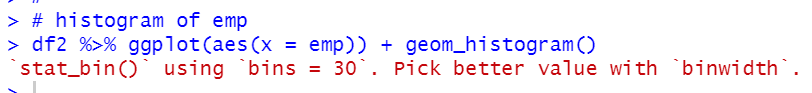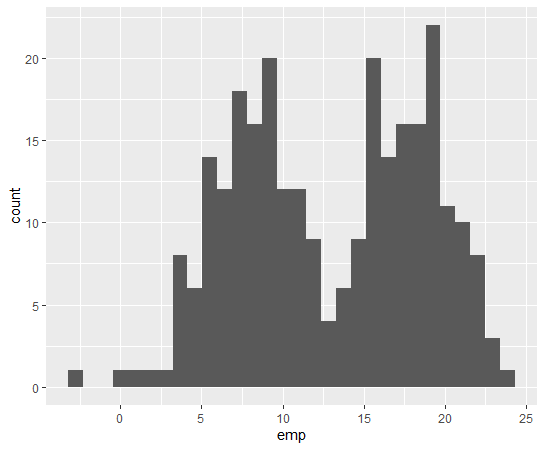We see double top distribution for emp.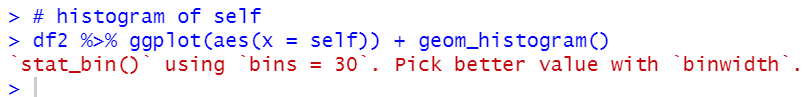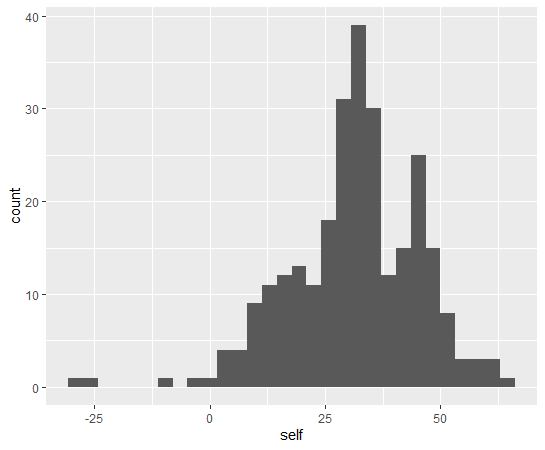self is single top distribution form.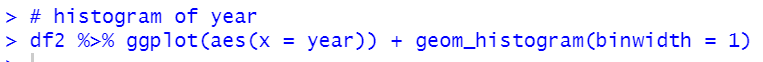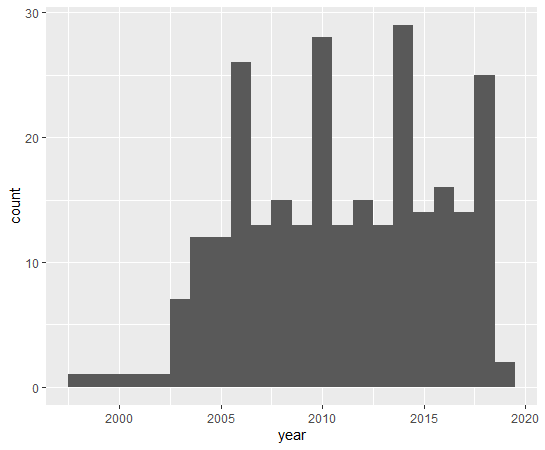Some year has more than 20 observations.

Next, let's see scatter plots using ggplot() + geom_point()

First, emp vs. self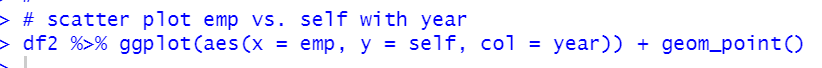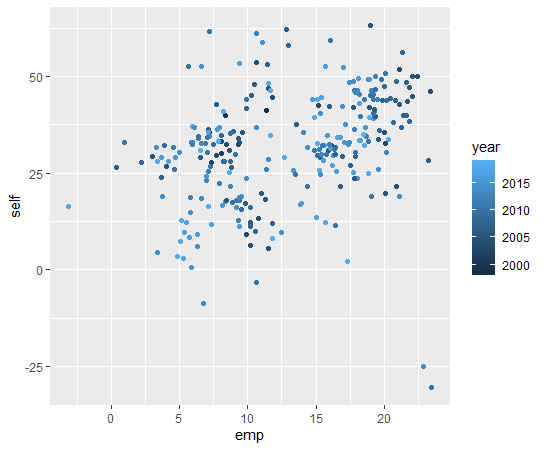There seems not strong linear relationship.

year vs. emp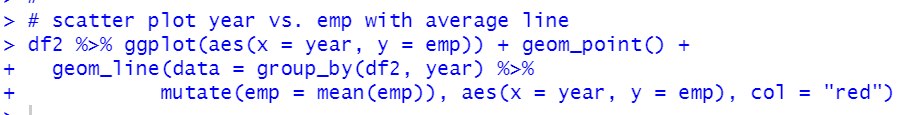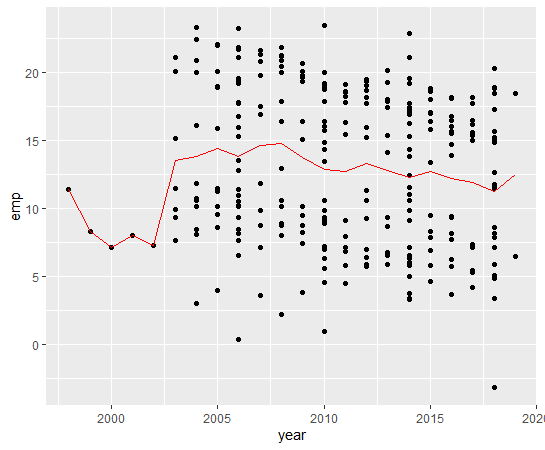I added year average line with using geom_line().

There seems slightly down trend.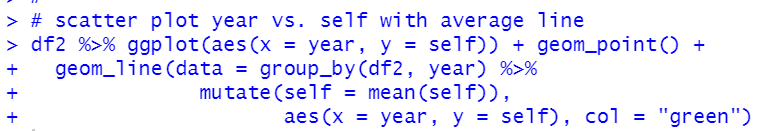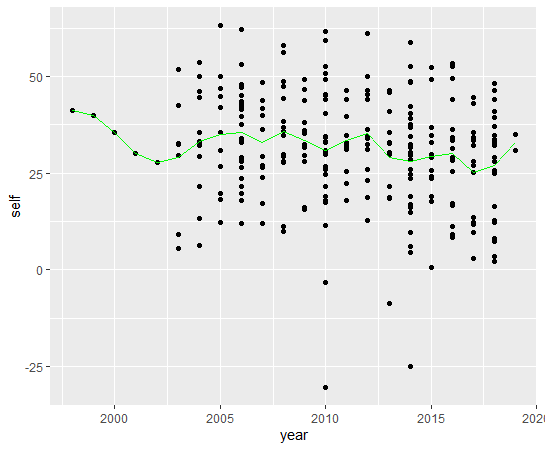Maybe, there seems weak downtrend.

Next, let's see country vs. emp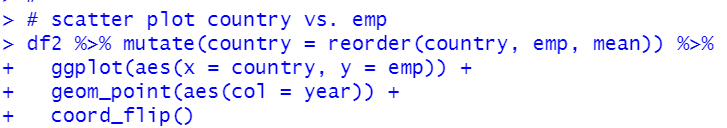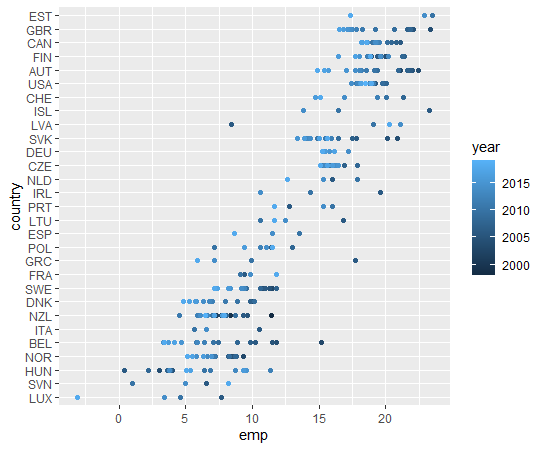I use reorder() function to re-order country by average emp.

We see many countries lowered gendar gap.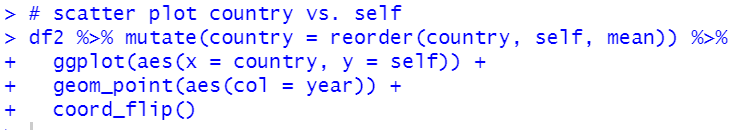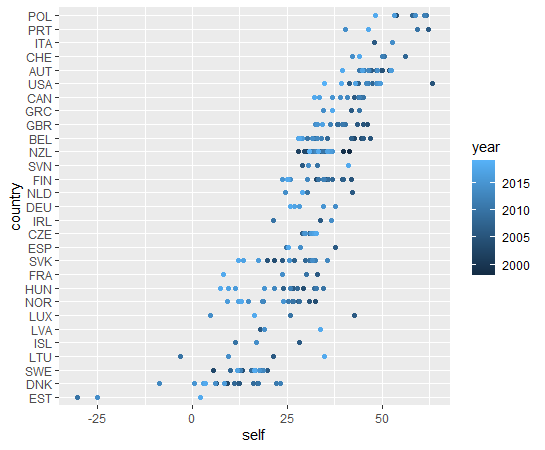For self, we see negative gender gap, it means female wage is higher than male age.

That's it. Thank you!

Next post is

To read from the 1st post,